Next: Historical Remarks Up: MAXWELL FIELDS: TRANSVERSE ELECTRIC Previous: The T.E.M. Field Equations

## Existence and Uniqueness of the Method of the 2+2 Split

Can an arbitrary vector potential be written in terms of four suitably chosen scalarsandso as to satisfy Eqs.(10), (12), and (15)? If the answer is yes'' then any e.m. field can be expressed in terms of these scalars, and one can claim that these scalars give a complete and equivalent description of the e.m. field. It turns out that this is indeed the case. In fact, the description is also unique. Indeed, given the vector potential, Eq.(7), there exist four unique scalars which are determined by this vector potential so as to satisfy Eqs.(10), (12), and (15). The determining equations, obtained by taking suitable derivatives, are
(19)
(20)
(21)
(22)

These equations guarantee the existence of the sought after scalar functionsand. Their uniqueness follows from their boundary conditions in the Euclidean-plane and their initial conditions in the Lorentzian
-plane. Consequently, Eqs.(19)-(22) together with Eqs.(10), (12), and (15) establish a one-to-one linear correspondence between the space of vector potentials and the space of four ordered scalars,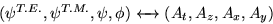Of the four scalars, three are gauge invariants, namely,, and the difference, a result made obvious by inspecting Eqs.(19)-(22).Next: Historical Remarks Up: MAXWELL FIELDS: TRANSVERSE ELECTRIC Previous: The T.E.M. Field Equations
Ulrich Gerlach 2001-10-09